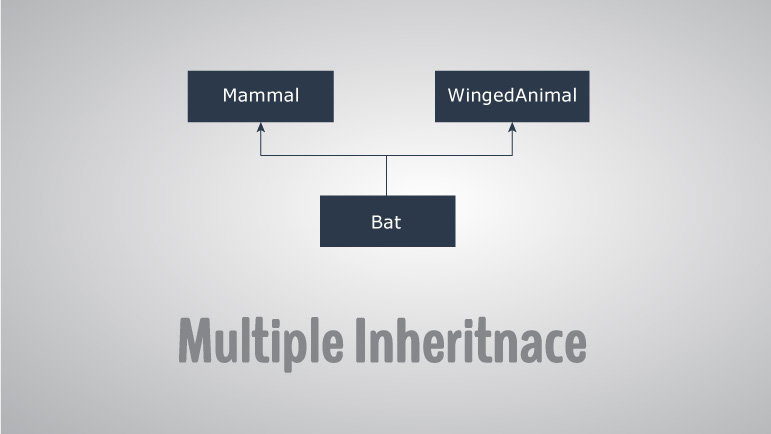# C++ Multiple, Multilevel and Hierarchical Inheritance

In this article, you will learn about different models of inheritance in C++ programming: Multiple, Multilevel and Hierarchical inheritance with examples.Inheritance is one of the core feature of an object-oriented programming language. It allows software developers to derive a new class from the existing class. The derived class inherits the features of the base class (existing class).

There are various models of inheritance in C++ programming.

## C++ Multilevel Inheritance

In C++ programming, not only you can derive a class from the base class but you can also derive a class from the derived class. This form of inheritance is known as multilevel inheritance.

```class A
{
... .. ...
};
class B: public A
{
... .. ...
};
class C: public B
{
... ... ...
};```

Here, class B is derived from the base class A and the class C is derived from the derived class B.

## Example 1: C++ Multilevel Inheritance

``````#include <iostream>
using namespace std;

class A
{
public:
void display()
{
cout<<"Base class content.";
}
};

class B : public A
{

};

class C : public B
{

};

int main()
{
C obj;
obj.display();
return 0;
}``````

Output

`Base class content.`

In this program, class C is derived from class B (which is derived from base class A).

The obj object of class C is defined in the `main()` function.

When the `display()` function is called, `display()` in class A is executed. It's because there is no `display()` function in class C and class B.

The compiler first looks for the `display()` function in class C. Since the function doesn't exist there, it looks for the function in class B (as C is derived from B).

The function also doesn't exist in class B, so the compiler looks for it in class A (as B is derived from A).

If `display()` function exists in C, the compiler overrides `display()` of class A (because of member function overriding).

## C++ Multiple Inheritance

In C++ programming, a class can be derived from more than one parents. For example: A class Bat is derived from base classes Mammal and WingedAnimal. It makes sense because bat is a mammal as well as a winged animal.## Example 2: Multiple Inheritance in C++ Programming

``````#include <iostream>
using namespace std;

class Mammal {
public:
Mammal()
{
cout << "Mammals can give direct birth." << endl;
}
};

class WingedAnimal {
public:
WingedAnimal()
{
cout << "Winged animal can flap." << endl;
}
};

class Bat: public Mammal, public WingedAnimal {

};

int main()
{
Bat b1;
return 0;
}
``````

Output

```Mammals can give direct birth.
Winged animal can flap.```

### Ambiguity in Multiple Inheritance

The most obvious problem with multiple inheritance occurs during function overriding.

Suppose, two base classes have a same function which is not overridden in derived class.

If you try to call the function using the object of the derived class, compiler shows error. It's because compiler doesn't know which function to call. For example,

``````class base1
{
public:
void someFunction( )
{ .... ... .... }
};
class base2
{
void someFunction( )
{ .... ... .... }
};
class derived : public base1, public base2
{

};

int main()
{
derived obj;

obj.someFunction() // Error!
}
```
```

This problem can be solved using scope resolution function to specify which function to class either base1 or base2

```int main()
{
obj.base1::someFunction( );  // Function of base1 class is called
obj.base2::someFunction();   // Function of base2 class is called.
}```

## C++ Hierarchical Inheritance

If more than one class is inherited from the base class, it's known as hierarchical inheritance. In hierarchical inheritance, all features that are common in child classes are included in the base class.

For example: Physics, Chemistry, Biology are derived from Science class.

### Syntax of Hierarchical Inheritance

```class base_class {
... .. ...
}

class first_derived_class: public base_class {
... .. ...
}

class second_derived_class: public base_class {
... .. ...
}

class third_derived_class: public base_class {
... .. ...
}```